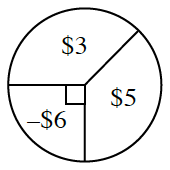### Home > GC > Chapter 11 > Lesson 11.1.5 > Problem11-69

11-69.

Multiple Choice: The probability of winning $3$ on the spinner below is equal to the chance of winning $5$. Find the expected value for one spin.

1. $\3.00$

1. $\4.50$

1. $\4.67$

1. $\6.00$

1. None of theseWhat fractions of the circle are the $3$ and $5$ sections?